Courses
Courses for Kids
Free study material
Free LIVE classes
More

# How to Write the Number 7?LIVE
Join Vedantu’s FREE Mastercalss

## Overview of the Number 7

Are you still bothered about how to make your child learn to identify the number 7? If so, you have now arrived at the appropriate spot, because this article has covered everything about the number 7, along with some worksheets and number 7 activities for preschool children. It uses engaging graphics that help children memorise the topic for a longer time. Solved examples and practice problems are also assigned to make learning easy. So, now let us begin with the topic.

## What is the Number 7?

The natural number 7 is the one that comes after 6 and before 8, making it a number, numeral, and digit. It is the only prime odd number which precedes the cube of a number, 2. Due to it being a prime number, its divisors are 1 and 7 only. This number is considered fortunate in several western countries.Number 7

## Number 7 With Objects

The digit count discussed in the article is seven. The objects are used to illustrate the number 7 visually using the images provided below. Parents and teachers may simply teach their children the number seven with the help of these illustrations.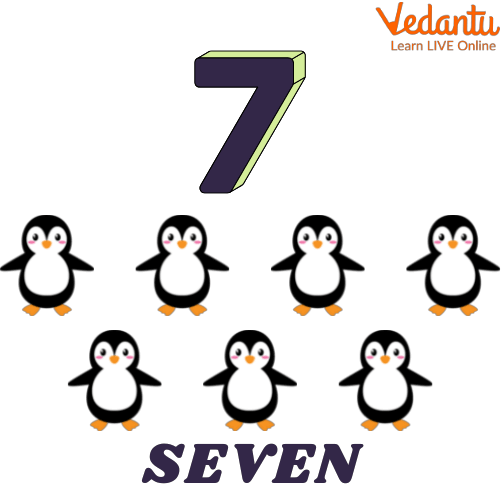Number 7 with Objects

## How to Write 7?

Children should regularly practise writing the number 7 by using counting to learn how to write the number 7. The worksheet provided below is based on the idea of the dotted and tracing phenomenon that helps kids learn how to write 7 quickly.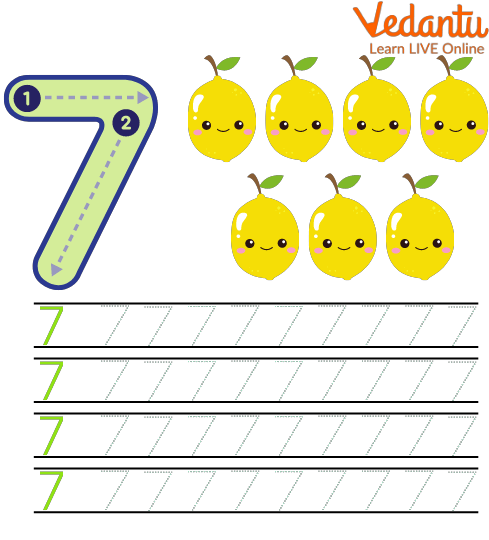How to Write 7?

## Writing Number 7 in Different Formats

The number 7 can be written in different forms, such as:

• In number names

The number 7 is represented as seven when written in number names.

• In ordinal form

In terms of ordinal form, the number 7 is written as seventh or 7th.

In roman numeral form, the number 7 is depicted by writing the symbol Vll.

In terms of the square root of a number, the number 7 is written as the square root of the number 49, i.e. $\sqrt{49}$

## Solved Examples

Q 1. The number seven lies between which two numbers?

Ans. As the number 7 is a counting number, therefore, it must lie between two numbers. Further, it is greater than the number 6 and less than the number 8. So, it lies between 6 and 8, where 6 is the predecessor of 7 while 8 is its successor.

Thus, the number 7 lies between 6 and 8.Number 7 between 6 and 8

Q 2. Represent the number 7 on the number line.

Ans. Follow the given steps to represent the number 7 on the number line:

• Draw a straight line of any length using the scale.

• Represent the integers on the number line.

• Mark the point where you get the number 7.

Thus, the number 7 is represented on the number line.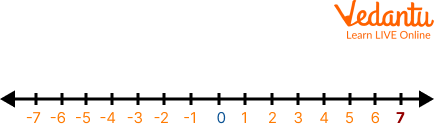7 on number line

## Practice Problems

By this time, children would have gained a distinction between 7 and the other numbers clearly, making it easier for the students to identify the number 7. Various worksheets and number 7 activities for preschool children are given below, which are based on the concept of counting and writing and circling the number 7. These number 7 activities for preschool children, would assist the tutors in evaluating the progress of the children.

Q 1. Count and write the number of characters shown in each line.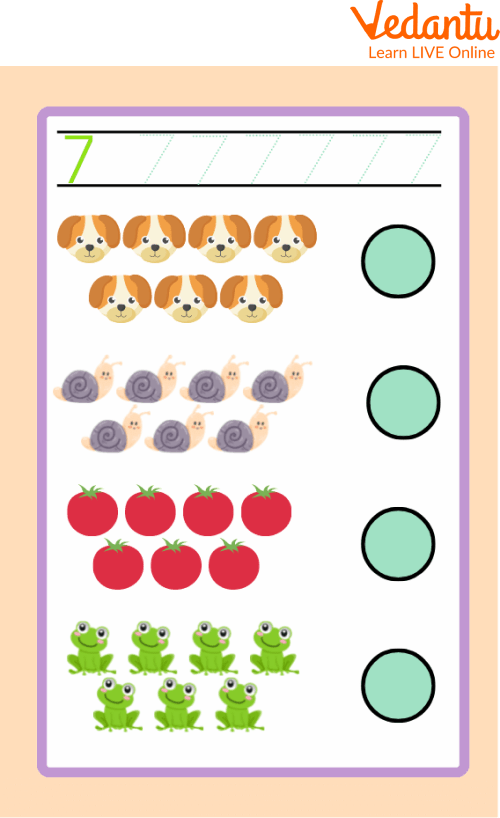Count and write 7

Q 2. How many 7’s are there in the image? Circle them.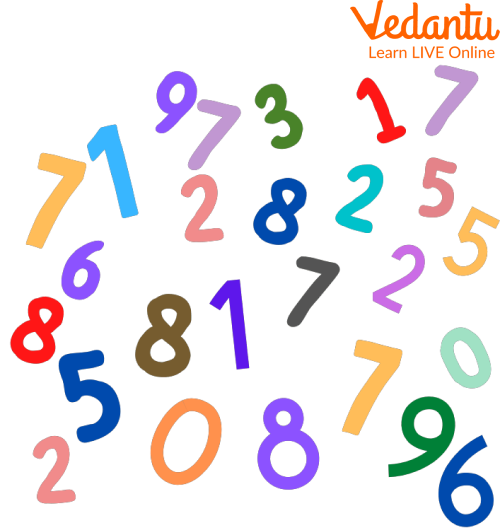Circle number 7

## Summary

To wrap up here with the topic of number 7. In memorising mathematics, numbers are the fundamental building blocks. This article covers every topic related to the number 7, along with how to write the number 7, using several graphics and easy language so that children will easily grasp the information being discussed here. Hoping that you find the article useful in learning the number 7 and enjoy reading it. Feel free to ask your doubts, if any, in the comments given below.

Last updated date: 02nd Oct 2023
Total views: 95.7k
Views today: 2.95k

## FAQs on How to Write the Number 7?

1. What is a number system?

A number system is a method of writing numbers; it is a way of mathematically representing a collection of numbers by using a consistent set of digits or other symbols. In several number systems, an identical set of symbols may symbolise various numbers.

2. What is special about the number 7?

The number 7 occurs a lot more frequently in nature, hence it is also known as the number of completion and perfection. There are seven oceans, seven continents, seven days in a week, seven colours in the rainbow, and seven neck vertebrae.

3. What do you mean by the counting and writing phenomenon?

The counting and writing phenomenon states that first, one should count the number of given objects and then write the number obtained as a result in the space provided aside. It serves as a useful tool for learning numbers easily.